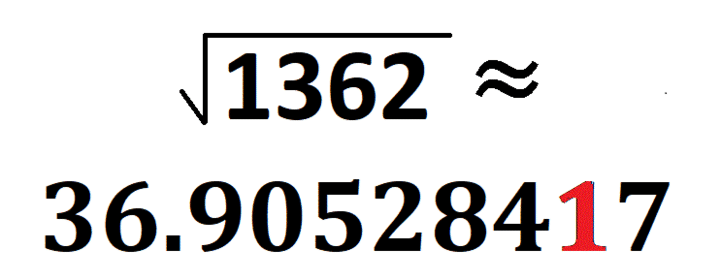# What Is Special about √1362

What is special about √1362? I don’t mean to sound like a Chevy commercial, but Just Look At It! I made this gif to show off √1362:make science GIFs like this at MakeaGif
1362 is the smallest number whose square root does that. Yeah, there have been some numbers that came close, but this one includes zero! Thank you OEIS.org for alerting me to that fact.
Here are a few more facts about the number 1362:
• 1362 is a composite number.
• Prime factorization: 1362 = 2 × 3 × 227
• 1362 has no exponents greater than 1 in its prime factorization, so √1362 cannot be simplified.
• The exponents in the prime factorization are 1, 1, and 1. Adding one to each exponent and multiplying we get (1 + 1)(1 + 1)(1 + 1) = 2 × 2 × 2 = 8. Therefore 1362 has exactly 8 factors.
• The factors of 1362 are outlined with their factor pair partners in the graphic below.
You can easily calculate a couple of monstrous Pythagorean triples that contain 1362:
1362-463760-463762 calculated from 2(681)(1), 681² – 1²,  681² + 1², and
1362-51520-51538 calculated from 2(227)(3), 227² – 3²,  227² + 3²